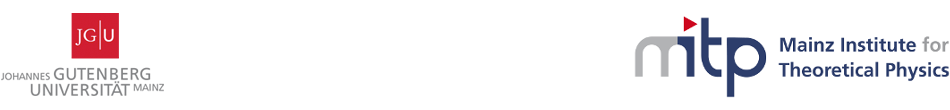#Geometry, Gravity and Supersymmetry

24-28 April 2017
Mainz Institute for Theoretical Physics, Johannes Gutenberg University
Europe/Berlin timezone

## Quaternionic Heisenberg group and Heterotic String Solutions with non-constant dilaton in dimensions 7 and 5

24 Apr 2017, 15:45
45m
02.430 (Mainz Institute for Theoretical Physics, Johannes Gutenberg University)

### 02.430

#### Mainz Institute for Theoretical Physics, Johannes Gutenberg University

Staudingerweg 9 / 2nd floor, 55128 Mainz

### Speaker

Prof. Stefan Ivanov (Sofia University)

### Description

Smooth solutions of the Strominger system with non vanishing flux, non-trivial instanton and non-constant dilaton based on the quaternionic Heisenberg group are constructed. It is shown that through appropriate contractions the solutions found in the $G_2$-heterotic case converge to the heterotic solutions on 6-dimensional inner non-K\"ahler spaces previously found and, moreover, to new heterotic solutions with non-constant dilaton in dimension 5. All the solutions satisfy the heterotic equations of motion up to the first order of \alpha^{\prime}.

### Primary author

Prof. Stefan Ivanov (Sofia University)

 Slides